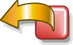A new and simpler primality test for Sophie-Germain numbers (q=2*p+1)

It is well known (Euler and Lagrange result) that if p=3 [mod 4] is prime, 2p+1 is also prime if and only if 2p+1 divides 2p-1.
I also found a same result for p=1[mod 4] prime. (Generalization of the Euler-Lagrange theorem and new primality tests).

I show here that it is possible to find a same simpler result, but with no condition for  the form of p.

If p>=5 is prime, q=2p+1 is also prime if and only if q divides 3p-1.

Demonstration :

1) If p and q=2p+1 are prime then q divides 3p+1 :
Indeed 32p-1 = (3p-1)*(3p+1)  and 32p-1 = 0 [mod q]  (Fermat th. for q).
If p>=5, p=6*a-1 or p=6*a+1. If p=6*a+1, q=12*a+3=0 [mod 3] ,  q not prime, therefore p=6*a-1 and q=12*a-1.
As q and 3 [mod 4] and 3=3 [mod 4], (3/q) = -(q/3)=-(-1/3)=1, therefore q divides 3p-1.

2) If p is prime and q=2p+1 divides 3p-1 then q is prime :
We use the "Well Known theorem" where n=h*pk+1 with h=2, p prime, n=q and k=1, it comes :
If q=2*p+1 and p>2, if there is an integer a such aq-1 = 1 [mod q] and gcd(a2-1,q) =1 then n is prime.
If a=3, 3p=1 [mod q] or 32p=3q-1 = 1 [mod q] and gcd(32-1=8,q) =1 , therefore q=2p+1 is prime.

Complements and consequences (soon ...)

Created by  Henri Lifchitz : January, 6  2000, last modification: January, 9 2000.